﻿ Graph of the Tangent Function

# GRAPH OF THE TANGENT FUNCTION

• PRACTICE (online exercises and printable worksheets)

This section discusses the graph of the tangent function (shown below).
For ease of reference, some material is repeated from the Trigonometric Functions.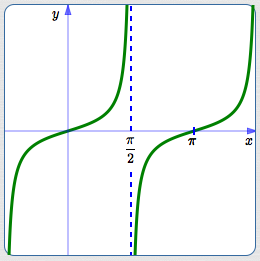graph of $y = \tan x$
(periodic with period $\,\pi\,$)

## The Tangent Function: Definition and Comments

• Let $\,t\,$ be a real number (with restrictions noted below).
Think of $\,t\,$, if desired, as the radian measure of an angle.
• By definition, $\displaystyle \tan t := \frac{\sin t}{\cos t}\,$.
• Using function notation, the number ‘$\,\tan t\,$’ is the output from the tangent function when the input is $\,t\,$.
In other words, ‘$\,\tan\,$’ is the name of the function; $\,t\,$ is the input; $\,\tan t\,$ is the corresponding output.
• Recall the convention: for multi-letter function names, parentheses are usually omitted from function notation.
That is, $\,\tan(t)\,$ is usually written without parentheses, as $\,\tan t\,$, when there is no confusion about order of operations.
• However, don't ever write something like ‘$\,\tan t + 2\,$’!
Clarify as either $\,(\tan t) + 2\,$ (better written as $\,2 + \tan t\,$) or $\,\tan(t+2)\,$.
• Pronounce ‘$\,\tan t\,$’ as ‘tangent of $\,t\,$’.
• The tangent function isn't defined where the cosine is zero; this happens at the terminal points $\,(0,1)\,$ and $\,(0,-1)\,$.
Thus, tangent is not defined for $\displaystyle \,t = \frac{k\pi}{2}\,$ for odd integers $\,k\,$:   $\,k = \ldots, -5, -3, -1, 1, 3, 5,\, \ldots\,$

## Where does the graph of the tangent function come from?

Recall that every real number is uniquely defined by its sign (plus or minus) and its size (distance from zero).

## Sign of the Tangent Function

The sign of $\,\tan t\,$ is easily determined from its definition as $\,\displaystyle\frac{\sin t}{\cos t}\,$:
• Quadrant I: cosine positive, sine positive, tangent positive
• Quadrant II: cosine negative, sine positive, tangent negative
• Quadrant III: cosine negative, sine negative, tangent positive
• Quadrant IV: cosine positive, sine negative, tangent negative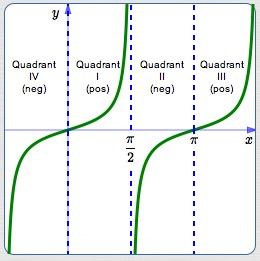Sign of the Tangent Function

## Size of the Tangent Function

The size of the tangent is best understood from its graphical significance
in the unit circle approach, as follows:
• View the real number $\,t\,$ as the radian measure of an angle.
Locate the angle $\,t\,$ in the unit circle.
The terminal point for $\,t\,$ is shown in black at right.
• Create an auxiliary (yellow) triangle (it overlaps the green triangle).
The base of the yellow triangle has length $\,1\,$.
The right-hand side is perpendicular to the $x$-axis and tangent to the circle.
• The green and (overlapping) yellow triangles are similar.
Why? They share the same central angle and they both have a right angle.
• This chart gives lengths of sides in the green and yellow triangles:

 bottom of triangle right side of triangle green triangle: $\cos t$ $\sin t$ yellow triangle: $1$ $y$(initially unknown)
• By similarity: $$\frac{\sin t}{\cos t} = \frac{y}{1}\,,\quad \text{ so }\quad \color{blue}{y = \frac{\sin t}{\cos t} := \tan t}$$
• Thus, the size of the tangent gives the length of a (particular) segment that is tangent to the unit circle. (The word ‘tangent’ derives from the Latin tangens, meaning ‘touching’.)
• Now, think about what happens to the length of this tangent segment as $\,t\,$ varies:
 As $\,t\,$ goes from to the size of $\,\tan t\,$ goes from to $-\frac{\pi}{2}$ $0$ $\infty$ $0$ $0$ $\frac{\pi}{2}$ $0$ $\infty$ $\frac{\pi}{2}$ $\pi$ $\infty$ $0$ $\pi$ $\frac{3\pi}{2}$ $0$ $\infty$ And so on!
• Put the size and sign together to get the graph of the tangent function.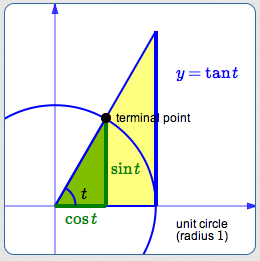Size of the Tangent FunctionVariations in Length
of the Tangent Segment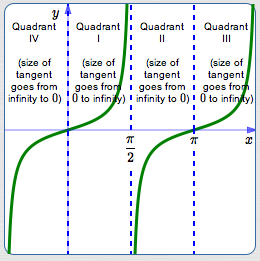Size of the Tangent Function

## Important Characteristics of the Graph of the Tangent Function

• The period of the tangent function is $\,\pi\,$:
$\tan(t+\pi) = \tan t\,$ for all real numbers $\,t\,$ in the domain.
• The domain of the tangent function excludes $\,\displaystyle\frac{\pi}{2} + k\pi\,$ for all integers $\,k\,$;
these are the values where the denominator of the tangent function (the cosine) is $\,0\,$.
• The range of the tangent function is the set of all real numbers;
the segment representing the tangent takes on all possible lengths from $\,0\,$ to $\,\infty\,$ (with both positive and negative signs).
• The tangent function has vertical asymptotes every place that it is not defined.
Let $\,c\,$ denote a real number that is not in the domain of the tangent function.
Then:
• as $\,t \rightarrow c^-\,$, $\,\tan t\rightarrow\infty\,$
• as $\,t \rightarrow c^+\,$, $\,\tan t\rightarrow -\infty\,$

## Tangent as Slope

There is an additional insight that comes from studying the diagram at right:
• The length of the green segment is $\,1\,$, since it is the radius of the unit circle.
• The slope of the red line is therefore: $$\frac{\text{rise}}{\text{run}} = \frac{\tan t}{1} = \tan t$$ The tangent gives the slope of the line!
• This works in all the quadrants:in the second quadrant,$\text{slope} = \tan t\,$is negativein the third quadrant, $\text{slope} = \tan t\,$ is positive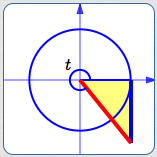in the fourth quadrant, $\text{slope} = \tan t\,$ is negative
• Summarizing: Start at the positive $x$-axis and lay off any angle $\,t\,$ (positive, negative, or zero). As long as the angle doesn't yield a vertical line (which has no slope), then $\,\tan t\,$ gives the slope of the resulting line. This is a useful fact in many applications.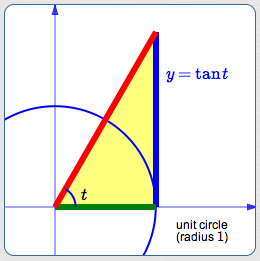Master the ideas from this section